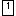# XOR/XNOR/Odd Parity/Even Parity Gate

 Library: Base Introduced: 2.0 Beta 1 for XOR/Odd/Even; 2.0 Beta 6 for XNOR Appearance:## Behavior

The XOR, XNOR, Even Parity, and Odd Parity gates each compute the respective function of the inputs, and emit the result on the output. The two-input truth table for the gates is the following.

xyXOR XNOROddEven
00 01 01
01 10 10
10 10 10
11 01 01
As you can see, the Odd Parity gate and the XOR gate behave identically with two inputs; similarly, the even parity gate and the XNOR gate behave identically. But if there are more than two specified inputs, the XOR gate will emit 1 only when there is exactly one 1 input, whereas the Odd Parity gate will emit 1 if there are an odd number of 1 inputs. The XNOR gate will emit 1 only when there is not exactly one 1 input, while the Even Parity gate will emit 1 if there are an even number of 1 inputs.

Any inputs that are unspecified (i.e., floating) are ignored. If all inputs are floating, then the output is floating, too. If any of the inputs are the error value (e.g., if conflicting values are coming into the same wire), then the output will be the error value, too.

The multi-bit versions of each gate will perform its one-bit transformation bitwise on its inputs.

Note: Many authorities contend that the shaped XOR gate's behavior should correspond to the odd parity gate, but there is not agreement on this point. Logisim's behavior for XOR gates is based on the IEEE 91 standard. It is also consistent with the intuitive meaning underlying the term exclusive or: A waiter asking whether you want a side dish of mashed potatoes, carrots, peas, or cole slaw will only accept one choice, not three, whatever some authorities may tell you. (I must admit, though, that I have not subjected this statement to a rigorous test.)

## Pins

West edge (inputs, bit width according to Bit Width attribute)
The inputs into the component. There will be as many of these as specified in the Number of Inputs attribute.

Note that if you are using shaped gates, the west side of XOR and XNOR gates will be curved. Nonetheless, the input pins are in a line. Logisim will draw short stubs illustrating this; and if you overshoot a stub, it will silently assume that you did not mean to overshoot it. In "printer view", these stubs will not be drawn unless they are connected to wires.

East edge (output, bit width according to Bit Width attribute)
The gate's output, whose value is computed based on the current inputs as described above.

## Attributes

Facing
The direction of the component (its output relative to its inputs).
Bit Width
The bit width of the component's inputs and outputs.
Gate Size
Determines whether to draw a wider or narrower version of the component. This does not affect the number of inputs, which is specified by the Number of Inputs attribute; however, if the number of inputs exceeds 3 (for a narrow component) or 5 (for a wide component), then the gate will be drawn with "wings" to be able to accommodate the number of inputs requested.
Number of Inputs
Determines how many pins to have for the component on its west side.

None.

## Text Tool Behavior

None.

Back to Library Reference